# NCERT Solutions For Class 11 Physics chapter-9 Mechanical Properties Of Solids

NCERT Solutions for class-11 Physics Chapter 9 Mechanical Properties of Solid is prepared by our senior and renowned teachers of Physics Wallah primary focus while solving these questions of class-11 in NCERT textbook, also do read theory of this Chapter 9 Mechanical Properties of Solid while going before solving the NCERT questions. You can download NCERT solution of all chapters from Physics Wallah in PDF.

### Chapter 9 Mechanical Properties of Solid

Question 1. A steel wire of length 4.7 m and cross-sectional area 3.0 × 10–5 m2 stretches by the same amount as a copper wire of length 3.5 m and cross-sectional area of 4.0 × 10–5 m2 under a given load. What is the ratio of the Young’s modulus of steel to that of copper?

Solution :
Length of the steel wire, L1 = 4.7 m
Area of cross-section of the steel wire, A1 = 3.0 × 10–5 m2
Length of the copper wire, L2 = 3.5 m
Area of cross-section of the copper wire, A2 = 4.0 × 10–5 m2
Change in length = ΔL1 = ΔL2 = ΔL
Force applied in both the cases = F
Young’s modulus of the steel wire:
Y1 = (F1 / A1) (L1 / ΔL1)
= (F / 3 X 10-5) (4.7 / ΔL)     ....(i)
Young’s modulus of the copper wire:
Y2 = (F2 / A2) (L2 / ΔL2)
= (F / 4 × 10-5) (3.5 / ΔL)     ....(ii)
Dividing (i) by (ii), we get:
Y1 / Y2  =  (4.7 × 4 × 10-5) / (3 × 10-5 × 3.5)
= 1.79 : 1
The ratio of Young’s modulus of steel to that of copper is 1.79 : 1.

Question2. Figure 9.11 shows the strain-stress curve for a given material. What are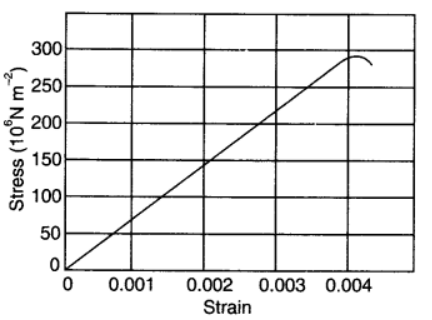a. Young’s modulus and

b. approximate yield strength for this material?

Solution :
(a) It is clear from the given graph that for stress 150 × 106 N/m2, strain is 0.002.
∴Young’s modulus, Y = Stress / Strain
= 150 × 106 / 0.002  =  7.5 × 1010 Nm-2
Hence, Young’s modulus for the given material is 7.5 ×1010 N/m2.

(b) The yield strength of a material is the maximum stress that the material can sustain without crossing the elastic limit.
It is clear from the given graph that the approximate yield strength of this material is 300 × 106 Nm/2 or 3 × 108 N/m2.

Question3. The stress-strain graphs for materials A and B are shown in Fig. 9.12.
The graphs are drawn to the same scale.

(a) Which of the materials has the greater Young’s modulus?
(b) Which of the two is the stronger material?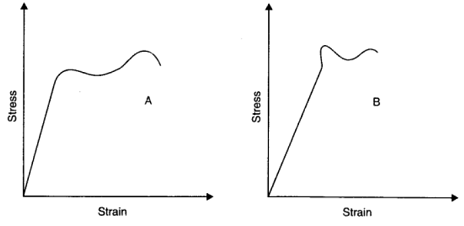Solution :
(a) From the two graphs we note that for a given strain, stress for A is more than that of B. Hence, Young's modulus (=stress/strain) is greater for A than that of B.

(b) A is stronger than B. Strength of a material is measured by the amount of stress required to cause fracture, corresponding to the point of fracture.

Question4. Read the following two statements below carefully and state, with reasons, if it is true or false.
(a) The Young’s modulus of rubber is greater than that of steel;
(b) The stretching of a coil is determined by its shear modulus.

Solution :
(a) False, because for given stress there is more strain in rubber than steel and modulus of elasticity is inversely proportional to strain.

(b) True, because the stretching of coil simply changes its shape without any change in the length of the wire used in the coil due to which shear modulus of elsticity is involved.

Question 5. Two wires of diameter 0.25 cm, one made of steel and the other made of brass are loaded as shown in Fig. 9.13. The unloaded length of steel wire is 1.5 m and that of brass wire is 1.0 m. Compute the elongations of the steel and the brass wires.

Solution :
Elongation of the steel wire = 1.49 × 10–4 m
Elongation of the brass wire = 1.3 × 10–4 m
Diameter of the wires, d = 0.25 m
Hence, the radius of the wires, r = d/2  =  0.125 cm
Length of the steel wire, L1 = 1.5 m
Length of the brass wire, L2 = 1.0 m
Total force exerted on the steel wire:
F1 = (4 + 6) g = 10 × 9.8 = 98 N
Young’s modulus for steel:
Y1 = (F1/A1) / (ΔL1 / L1)
Where,
ΔL1 = Change in the length of the steel wire
A1 = Area of cross-section of the steel wire = πr12
Young’s modulus of steel, Y1 = 2.0 × 1011 Pa
∴ ΔL1 = F1  × L1 / (A1  × Y1)
= (98  × 1.5) /[ π(0.125  × 10-2)2  × 2  × 1011]   =   1.49  × 10-4 m

Total force on the brass wire:
F2 = 6 × 9.8 = 58.8 N
Young’s modulus for brass:
Y2 = (F2/A2)/ (ΔL2 / L2)
Where,
ΔL2 = Change in the length of the brass wire
A1 = Area of cross-section of the brass wire = πr12
∴ ΔL2 = F2  × L2 / (A2  × Y2)
= (58.8 X 1) / [ (π  × (0.125  × 10-2)2  × (0.91  × 1011)] = 1.3  × 10-4 m
Elongation of the steel wire = 1.49 × 10–4 m
Elongation of the brass wire = 1.3 × 10–4 m.

Question 6. The edge of an aluminium cube is 10 cm long. One face of the cube is firmly fixed to a vertical wall. A mass of 100 kg is then attached to the opposite face of the cube. The shear modulus of aluminium is 25 GPa. What is the vertical deflection of this face?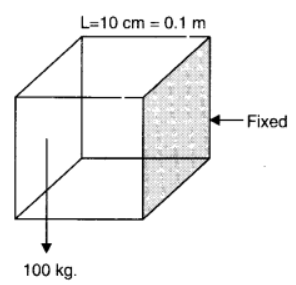Solution :
Edge of the aluminium cube, L = 10 cm = 0.1 m
The mass attached to the cube, m = 100 kg
Shear modulus (η) of aluminium = 25 GPa = 25 × 109 Pa
Shear modulus, η = Shear stress / Shear strain  =  (F/A) / (L/ΔL)
Where,
F = Applied force = mg = 100 × 9.8 = 980 N
A = Area of one of the faces of the cube = 0.1 × 0.1 = 0.01 m2
ΔL = Vertical deflection of the cube
∴ ΔL = FL / Aη
= 980 × 0.1/ [ 10-2 × (25 × 109) ]
= 3.92 × 10–7 m
The vertical deflection of this face of the cube is 3.92 ×10–7 m.

Question 7. Four identical hollow cylindrical columns of mild steel support a big structure of mass 50,000 kg. The inner and outer radii of each column are 30 cm and 60 cm respectively. Assuming the load distribution to be uniform, calculate the compressional strain of each column.< /p>

Solution :
Mass of the big structure, M = 50,000 kg
Inner radius of the column, r = 30 cm = 0.3 m
Outer radius of the column, R = 60 cm = 0.6 m
Young’s modulus of steel, Y = 2 × 1011 Pa
Total force exerted, F = Mg = 50000 × 9.8 N
Stress = Force exerted on a single column = 50000 × 9.8 / 4  =  122500 N
Young’s modulus, Y = Stress / Strain
Strain = (F/A) / Y
Where,
Area, A = π (R2 – r2) = π ((0.6)2 – (0.3)2)
Strain = 122500/ [ π ((0.6)2 – (0.3)2) × 2 × 1011 ]  =  7.22 × 10-7
Hence, the compressional strain of each column is 7.22 × 10–7.

Question8. A piece of copper having a rectangular cross-section of 15.2 mm × 19.1 mm is pulled in tension with 44,500 N force, producing only elastic deformation. Calculate the resulting strain?

Solution :
Length of the piece of copper, l = 19.1 mm = 19.1 × 10–3 m
Breadth of the piece of copper, b = 15.2 mm = 15.2 × 10–3 m
Area of the copper piece:
A = l × b
= 19.1 × 10–3 × 15.2 × 10–3
= 2.9 × 10–4 m2
Tension force applied on the piece of copper, F = 44500 N
Modulus of elasticity of copper, η = 42 × 109 N/m2
Modulus of elasticity, η = Stress / Strain
= (F/A) / Strain
∴ Strain = F / Aη
= 44500/ (2.9 × 10-4 × 42 × 109)
= 3.65 × 10–3.

Question 9. A steel cable with a radius of 1.5 cm supports a chairlift at a ski area. If the maximum stress is not to exceed 108 N m–2, what is the maximum load the cable can support?

Solution :
Radius of the steel cable, r = 1.5 cm = 0.015 m
Maximum allowable stress = 108 N m–2
Maximum stress = Maximum force / Area of cross-section
∴ Maximum force = Maximum stress × Area of cross-section
= 108 × π (0.015)2
= 7.065 × 104 N
Hence, the cable can support the maximum load of 7.065 × 104 N.

Question 10. A rigid bar of mass 15 kg is supported symmetrically by three wires each 2.0 m long. Those at each end are of copper and the middle one is of iron. Determine the ratio of their diameters if each is to have the same tension.

Solution :
The tension force acting on each wire is the same. Thus, the extension in each case is the same. Since the wires are of the same length, the strain will also be the same.
The relation for Young’s modulus is given as:
Y = Stress / Strain
= (F/A)/ Strain  =  (4F/πd2)/ Strain     ....(i)
Where,
F = Tension force
A = Area of cross-section
d = Diameter of the wire
It can be inferred from equation (i) that Y ∝ (1/d2)
Young’s modulus for iron, Y1 = 190 × 109 Pa
Diameter of the iron wire = d1
Young’s modulus for copper, Y2 = 120 × 109 Pa
Diameter of the copper wire = d2
Therefore, the ratio of their diameters is given as: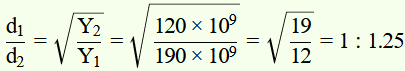Question 11. A 14.5 kg mass, fastened to the end of a steel wire of unstretched length 1.0 m, is whirled in a vertical circle with an angular velocity of 2 rev/s at the bottom of the circle. The cross-sectional area of the wire is 0.065 cm2. Calculate the elongation of the wire when the mass is at the lowest point of its path.

Solution :
Mass, m = 14.5 kg
Length of the steel wire, l = 1.0 m
Angular velocity, ω = 2 rev/s = 2 × 2π rad/s = 12.56 rad/s
Cross-sectional area of the wire, a = 0.065 cm2 = 0.065 × 10-4 m2
Let Δl be the elongation of the wire when the mass is at the lowest point of its path.
When the mass is placed at the position of the vertical circle, the total force on the mass is:
F = mg + mlω2
= 14.5 × 9.8 + 14.5 × 1 × (12.56)2
= 2429.53 N
Young's modulus = Strss / Strain
Y = (F/A) / (∆l/l)
∴ ∆l = Fl / AY
Young’s modulus for steel = 2 × 1011 Pa
∆l = 2429.53 × 1 / (0.065 × 10-4 × 2 × 1011)   =   1.87 × 10-3 m
Hence, the elongation of the wire is 1.87 × 10–3 m.

Question 12. Compute the bulk modulus of water from the following data: Initial volume = 100.0 litre, Pressure increase = 100.0 atm (1 atm = 1.013 × 105 Pa), Final volume = 100.5 litre. Compare the bulk modulus of water with that of air (at constant temperature). Explain in simple terms why the ratio is so large.

Solution :
Initial volume, V1 = 100.0l = 100.0 × 10 –3 m3
Final volume, V2 = 100.5 l = 100.5 ×10 –3 m3
Increase in volume, ΔV = V2 – V1 = 0.5 × 10–3 m3
Increase in pressure, Δp = 100.0 atm = 100 × 1.013 × 105 Pa
Bulk modulus = Δp/ (ΔV/V1)  =  Δp × V1 / ΔV
= 100 × 1.013 × 105 × 100 × 10-3 / (0.5 × 10-3)
= 2.026 × 109 Pa
Bulk modulus of air = 1 × 105 Pa
∴ Bulk modulus of water / Bulk modulus of air  =  2.026 × 109/ (1 × 105)  =  2.026 × 104
This ratio is very high because air is more compressible than water.

Question13. What is the density of water at a depth where pressure is 80.0 atm, given that its density at the surface is 1.03 × 103 kg m–3?

Solution :
Let the given depth be h.
Pressure at the given depth, p = 80.0 atm = 80 × 1.01 × 105 Pa
Density of water at the surface, ρ1 = 1.03 × 103 kg m–3
Let ρ2 be the density of water at the depth h.
Let V1 be the volume of water of mass m at the surface.
Let V2 be the volume of water of mass m at the depth h.
Let ΔV be the change in volume.
ΔV = V1 - V2
= m [ (1/ρ1) - (1/ρ2) ]
∴ Volumetric strain = ΔV / V1
= m [ (1/ρ1) - (1/ρ2) ] × (ρ1 / m)
ΔV / V1 = 1 - (ρ12)     ......(i)
Bulk modulus, B = pV1 / ΔV
ΔV / V1 = p / B
Compressibility of water = (1/B) = 45.8 × 10-11 Pa-1
∴ ΔV / V1 = 80 × 1.013 × 105 × 45.8 × 10-11  =  3.71 × 10-3    ....(ii)
For equations (i) and (ii), we get:
1 - (ρ12)   =   3.71 × 10-3
ρ2 = 1.03 × 103/ [ 1 - (3.71 × 10-3) ]
= 1.034 × 103 kg m-3
Therefore, the density of water at the given depth (h) is 1.034 × 103 kg m–3.

Question14. Compute the fractional change in volume of a glass slab, when subjected to a hydraulic pressure of 10 atm.

Solution :
Hydraulic pressure exerted on the glass slab, p = 10 atm = 10 × 1.013 × 105 Pa
Bulk modulus of glass, B = 37 × 109 Nm–2
Bulk modulus, B = p / (∆V/V)
Where,
∆V/V = Fractional change in volume
∴ ∆V/V = p / B
= 10 × 1.013 × 105 / (37 × 109)
= 2.73 × 10-5
Hence, the fractional change in the volume of the glass slab is 2.73 × 10–5.

Question15. Determine the volume contraction of a solid copper cube, 10 cm on an edge, when subjected to a hydraulic pressure of 7.0 ×106 Pa.

Solution :
Length of an edge of the solid copper cube, l = 10 cm = 0.1 m
Hydraulic pressure, p = 7.0 × 106 Pa
Bulk modulus of copper, B = 140 × 109 Pa
Bulk modulus, B = p / (∆V/V)
Where,
∆V/V = Volumetric strain
ΔV = Change in volume
V = Original volume.
ΔV = pV / B
Original volume of the cube, V = l3
∴ ΔV = pl3 / B
= 7 × 106 × (0.1)3 / (140 × 109)
= 5 × 10-8 m3  =  5 × 10-2 cm-3
Therefore, the volume contraction of the solid copper cube is 5 × 10–2 cm–3.

Question16. How much should the pressure on a litre of water be changed to compress it by 0.10%?

Solution :
Volume of water, V = 1 L
It is given that water is to be compressed by 0.10%.
∴ Fractional change, ∆V / V = 0.1 / (100 × 1)  =  10-3
Bulk modulus, B = ρ / (∆V/V)
ρ = B × (∆V/V)
Bulk modulus of water, B = 2.2 × 109 Nm-2
ρ = 2.2 × 109 × 10-3  =  2.2 × 106 Nm-2
Therefore, the pressure on water should be 2.2 ×106 Nm–2.

Question17. Anvils made of single crystals of diamond, with the shape as shown in Fig. 9.14, are used to investigate behaviour of materials under very high pressures. Flat faces at the narrow end of the anvil have a diameter of 0.50 mm, and the wide ends are subjected to a compressional force of 50,000 N. What is the pressure at the tip of the anvil?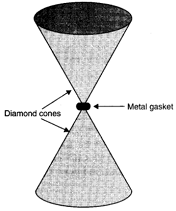Solution :
Diameter of the cones at the narrow ends, d = 0.50 mm = 0.5 × 10–3 m
Radius, r = d/2  =  0.25 × 10-3 m
Compressional force, F = 50000 N
Pressure at the tip of the anvil:
P = Force / Area  =  50000/ π(0.25 × 10-3)2
= 2.55  × 1011 Pa
Therefore, the pressure at the tip of the anvil is 2.55 × 1011 Pa.

Question 18. A rod of length 1.05 m having negligible mass is supported at its ends by two wires of steel (wire A) and aluminium (wire B) of equal lengths as shown in Fig. 9.15. The cross-sectional areas of wires A and B are 1.0 mm2 and 2.0 mm2, respectively. At what point along the rod should a mass m be suspended in order to produce (a) equal stresses and (b) equal strains in both steel and aluminium wires.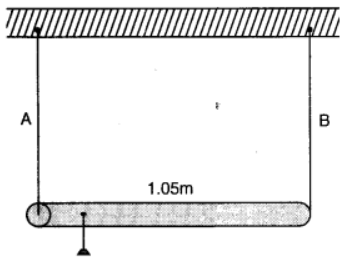Solution :
Cross-sectional area of wire A, a1 = 1.0 mm2 = 1.0 × 10–6 m2
Cross-sectional area of wire B, a2 = 2.0 mm2 = 2.0 × 10–6 m2
Young’s modulus for steel, Y1 = 2 × 1011 Nm–2
Young’s modulus for aluminium, Y2 = 7.0 ×1010 Nm–2

(a) Let a small mass m be suspended to the rod at a distance y from the end where wire A is attached.
Stress in the wire = Force / Area  =  F / a
If the two wires have equal stresses, then:
F1 / a1  =  F2 / a2
Where,
F1 = Force exerted on the steel wire
F2 = Force exerted on the aluminum wire
F1 / F2 = a1 / a2  =  1 / 2    ....(i)
The situation is shown in the following figure: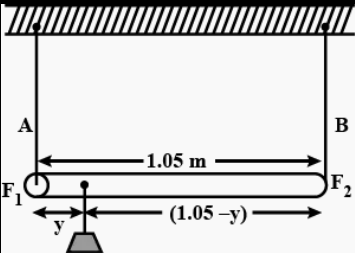Taking torque about the point of suspension, we have:
F1y = F2 (1.05 - y)
F1 / F2 = (1.05 - y) / y    ......(ii)
Using equations (i) and (ii), we can write:
(1.05 - y) / y  = 1 / 2
2(1.05 - y)  =  y
y = 0.7 m
In order to produce an equal stress in the two wires, the mass should be suspended at a distance of 0.7 m from the end where wire A is attached.

(b) Young's modulus = Stress / Strain
Strain = Stress / Young's modulus  =  (F/a) / Y
If the strain in the two wires is equal, then:
(F1/a1)/ Y1  =  (F2/a2)/ Y2
F1 / F2 = a1Y1 / a2Y2
a1 / a2 = 1/2
F1 / F2 = (1 / 2) (2 × 1011 / 7 × 1010)  =  10 / 7      .......(iii)
Taking torque about the point where mass m, is suspended at a distance y1 from the side where wire A attached, we get:
F1y1 = F2 (1.05 – y1)
F1 / F2  =  (1.05 - y1) / y1    ....(iii)
Using equations (iii) and (iv), we get:
(1.05 - y1) / y1  =  10 / 7
7(1.05 - y1)  =  10y1
y1 = 0.432 m
In order to produce an equal strain in the two wires, the mass should be suspended at a distance of 0.432 m from the end where wire A is attached.

Question 19. A mild steel wire of length 1.0 m and cross-sectional area 0.50 × 10–2 cm2 is stretched, well within its elastic limit, horizontally between two pillars. A mass of 100 g is suspended from the mid-point of the wire. Calculate the depression at the midpoint.

Solution :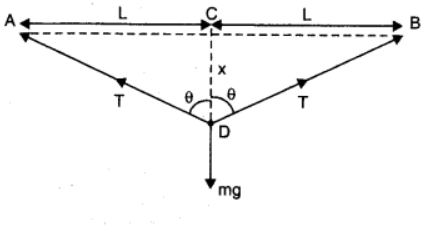From the above figure,

Let x be the depression at the mid point

i.e. CD = x.

In fig.,

AC= CB = l = 0.5 m ;

m = 100 g = 0.100 Kg

AD= BD = (l2 + x2)1/2

Increase in length, ∆l = AD + DB - AB = 2AD - AB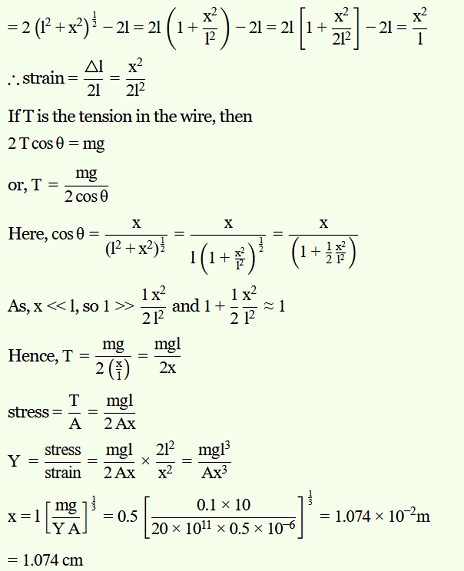Question 20. Two strips of metal are riveted together at their ends by four rivets, each of diameter 6.0 mm. What is the maximum tension that can be exerted by the riveted strip if the shearing stress on the rivet is not to exceed 6.9 × 107 Pa? Assume that each rivet is to carry one quarter of the load.

Solution :
Diameter of the metal strip, d = 6.0 mm = 6.0 × 10–3 m
Radius, r = d/2 = 3 × 10-3 m
Maximum shearing stress = 6.9 × 107 Pa
Maximum stress = Manimum load or force / Area
Maximum force = Maximum stress × Area
= 6.9 × 107 × π × (r) 2
= 6.9 × 107 × π × (3 ×10–3)2
= 1949.94 N
Each rivet carries one quarter of the load.
∴ Maximum tension on each rivet = 4 × 1949.94 = 7799.76 N.

Question 21. The Marina trench is located in the Pacific Ocean, and at one place it is nearly eleven km beneath the surface of water. The water pressure at the bottom of the trench is about 1.1 × 108 Pa. A steel ball of initial volume 0.32 m3 is dropped into the ocean and falls to the bottom of the trench. What is the change in the volume of the ball when it reaches to the bottom?

Solution :
Water pressure at the bottom, p = 1.1 × 108 Pa
Initial volume of the steel ball, V = 0.32 m3
Bulk modulus of steel, B = 1.6 × 1011 Nm–2
The ball falls at the bottom of the Pacific Ocean, which is 11 km beneath the surface.
Let the change in the volume of the ball on reaching the bottom of the trench be ΔV.
Bulk modulus, B = p / (∆V/V)
∆V  =  B / pV
= 1.1 × 108 × 0.32 / (1.6 × 1011 )  =  2.2 × 10-4 m3
Therefore, the change in volume of the ball on reaching the bottom of the trench is 2.2 × 10–4 m3.